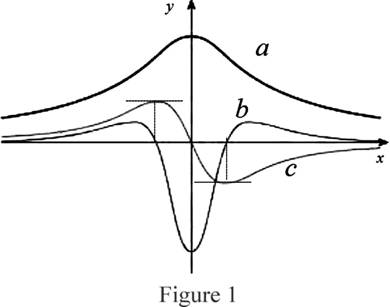# The curves f , f ′ and f ″ on given with proper explanation.### Single Variable Calculus: Concepts...

4th Edition
James Stewart
Publisher: Cengage Learning
ISBN: 9781337687805### Single Variable Calculus: Concepts...

4th Edition
James Stewart
Publisher: Cengage Learning
ISBN: 9781337687805

#### Solutions

Chapter 2, Problem 40RE
To determine

## To Identify: The curves f,f′ and f″ on given with proper explanation.

Expert Solution

In the given graph, a=f,b=f and c=f.

### Explanation of Solution

Graph:

The given graph is shown as in Figure 1,Observation:

From the Figure 1, it is observed that the point b(x)=0 is the same point where the graph of c has horizontal tangents.

Recall that the derivative of a function is zero where the function has a horizontal tangent.

Therefore, b(x) is the derivative of the graph c(x). That is, c(x)=b(x).

Observe the graph of a and c carefully.

The slope of the curve is positive for x<0 and the slope is negative for x>0. c is the only curve which is positive or x<0 and negative for x>0.

Therefore, c(x) is the derivative of the graph a(x). That is, a(x)=c(x).

Use the above information a(x)=c(x) and c(x)=b(x) to concluded that a(x)=b(x).

Thus, a=f, b=f and c=f.

### Have a homework question?

Subscribe to bartleby learn! Ask subject matter experts 30 homework questions each month. Plus, you’ll have access to millions of step-by-step textbook answers!